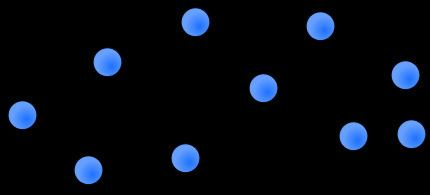# Weighted network

Updated on
Covid-19A weighted network is a network where the ties among nodes have weights assigned to them. A network is a system whose elements are somehow connected (Wasserman and Faust, 1994). The elements of a system are represented as nodes (also known as actors or vertices) and the connections among interacting elements are known as ties, edges, arcs, or links. The nodes might be neurons, individuals, groups, organisations, airports, or even countries, whereas ties can take the form of friendship, communication, collaboration, alliance, flow, or trade, to name a few.

## Contents

In a number of real-world networks, not all ties in a network have the same capacity. In fact, ties are often associated with weights that differentiate them in terms of their strength, intensity, or capacity (Barrat et al., 2004) and Horvath (2011). On the one hand, Mark Granovetter (1973) argued that the strength of social relationships in social networks is a function of their duration, emotional intensity, intimacy, and exchange of services. On the other, for non-social networks, weights often refer to the function performed by ties, e.g., the carbon flow (mg/m²/day) between species in food webs (Luczkowich et al., 2003), the number of synapses and gap junctions in neural networks (Watts and Strogatz, 1998), or the amount of traffic flowing along connections in transportation networks (Opsahl et al., 2008).

By recording the strength of ties, a weighted network can be created (also known as a valued network). Below is an example of such a network (weights can also be visualized by giving edges different widths):

Weighted networks are also widely used in genomic and systems biologic applications. (Horvath, 2011). For example, weighted gene co-expression network analysis (WGCNA) is often used for constructing a weighted network among genes (or gene products) based on gene expression (e.g. microarray) data (Zhang and Horvath 2005). More generally, weighted correlation networks can be defined by soft-thresholding the pairwise correlations among variables (e.g. gene measurements).

## Measures for weighted networks

Although weighted networks are more difficult to analyse than if ties were simply present or absent, a number of network measures has been proposed for weighted networks:

• Node strength: The sum of weights attached to ties belonging to a node (Barrat et al., 2004)
• Closeness: Redefined by using Dijkstra's distance algorithm (Newman, 2001)
• Betweenness: Redefined by using Dijkstra's distance algorithm (Brandes, 2001) (details)
• The clustering coefficient (global): Redefined by using a triplet value (Opsahl and Panzarasa, 2009)
• The clustering coefficient (local): Redefined by using a triplet value (Barrat et al., 2004) or using an algebraic formula (Zhang and Horvath 2005)
• A theoretical advantage of weighted networks is that they allow one to derive relationships among different network measures (also known as network concepts, statistics or indices. For example, Dong and Horvath (2007) show that simple relationships among network measures can be derived in clusters of nodes (modules) in weighted networks. For weighted correlation networks, one can use the angular interpretation of correlations to provide a geometric interpretation of network theoretic concepts and to derive unexpected relationships among them Horvath and Dong (2008)

## Software for analysing weighted networks

There are a number of software packages that can analyse weighted networks, see Social network analysis software. Among these are the proprietary software UCINET and the open-source package tnet.

The WGCNA R package implements functions for constructing and analyzing weighted networks in particular weighted correlation networks.

## References

Similar Topics
The Worlds Greatest Athlete
Innocent Thing
Lee Peltier
Topics